# Introduction

DiffusionRgqd is an R package for performing inference and analysis on diffusion processes, a class of continuous time, continuous state Markov processes often used in fields such as physics and finance. Diffusion processes encompass many famous stochastic processes encountered in graduate and post-graduate level statistics courses, such as Brownian motion and the Ornstein-Uhlenbeck process process. Although diffusion processes are extremely useful modelling tools, performing analysis on models that are even slightly more complicated than the aforementioned models quickly become difficult. This is due in part to the fact that most diffusion models do not have analytically tractable dynamics. DiffusionRgqd provides tools for performing such analysis on a class of non-linear, time inhomogeneous diffusion processes, making it possible to access non-linear diffusion processes with complicated time-inhomogeneous structures, without the need to perform any complicated mathematics beyond specifying the model of interest and peripheral parameters to the problem.

DiffusionRgqd is built around a class of diffusion processes with quadratic drift and diffusion specifications. That is, for an SDE: $dX_t = \mu(X_t,t)dt +\sigma(X_t,t)dB_t$ where $$B_t$$ is standard Brownian motion, the drift and diffusion terms assume at most second order non-linearity: $\mu(X_t,t) = G_0(t)+G_1(t)X_t+G_2(t)X_t^2$ and $\sigma^2(X_t,t) = Q_0(t)+Q_1(t)X_t+Q_2(t)X_t^2.$ The purpose of this restriction is to provide a mathematical ‘sandbox’ within which we can calculate very accurate approximations to the transitional density of a diffusion process whilst maximizing freedom with respect to specifying time-inhomogeneous components. That is, within this framework, although we have limited the complexity of state-dependence in the drift and diffusion of the model, one has nearly complete freedom of specification for time-inhomogeneous coefficients of the model (i.e., G0(t), Q1(t) etc.).

# Interface

Due to the freedom that the GQD framework permits, we have designed the interface of the package around a functional input system, where the user specifies the coefficients of the GQD as normal R-functions. For example, the SDE

$dX_t = -\sin(2\pi t)X_tdt +dB_t$

can be written in R-syntax in terms of its GQD-coefficients as

G1=function(t){-sin(2*pi*t)}
Q0=function(t){1}

The idea is to provide a grammatical link between the lexical structure of a generalized diffusion model in written language, to the R language. Routines in the DiffusionRgqd package thus operate by searching the current R workspace for pre-defined coefficient names after which it subsequently constructs the appropriate algorithm in order to perform analysis. For example, in the case of scalar GQDs, recognized function names are tabled below.

Coefficient R-syntax Coefficient R-syntax
$$G_0(t)$$ G0=function(t){} $$Q_0(t)$$ Q0=function(t){}
$$G_1(t)$$ G1=function(t){} $$Q_1(t)$$ Q1=function(t){}
$$G_2(t)$$ G2=function(t){} $$Q_2(t)$$ Q2=function(t){}

# Brownian motion with drift

Consider a stochastic differential equation

$dX_t = \mu dt +\sigma dB_t$

where $$B_t$$ is standard Brownian motion. Then the distribution of the process $$X_t$$ at time $$t$$, given that it started in state $$X_s$$ at time $$s$$ is $$\mbox{Normal}(X_t,X_s+\mu(t-s),\sigma^2(t-s))$$. For example:

Xs <- 0                 # Initial state
Xt <- seq(-3/2,3/2,1/50)# Possible future states
s  <- 0                 # Starting time
t  <- 1                 # Final time
mu    <- 0.5            # Drift parameter
sigma <- 0.25           # Diffusion coefficient

# True transition density
plot(dnorm(Xt, Xs + mu*(t-s), sigma*sqrt(t-s))~Xt, main = 'Transition density', type = 'l')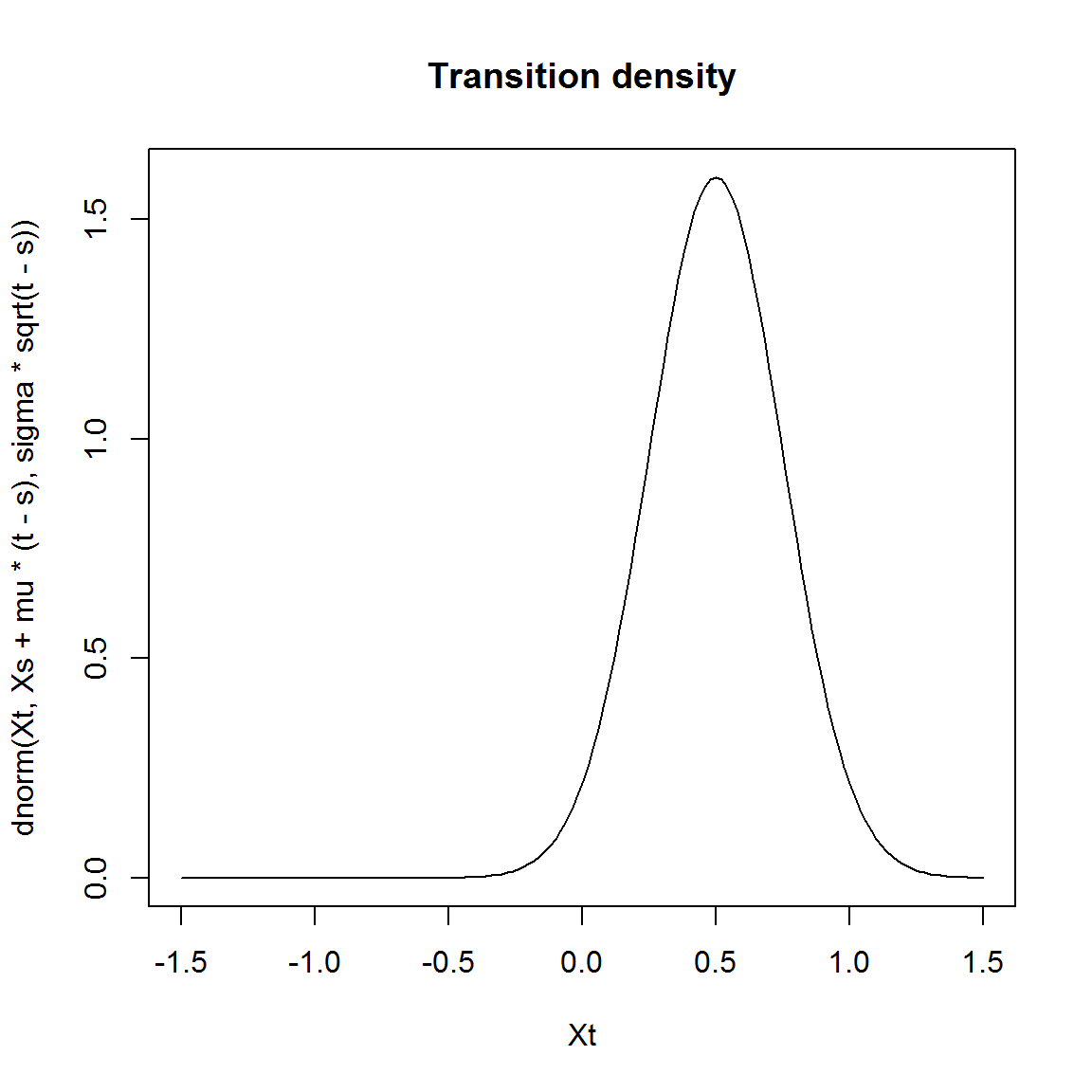Using DiffusionRgqd we can replicate the transition density of Brownian motion with drift by using the GQD-framework:

library(DiffusionRgqd)

# Remove any existing coefficients:
GQD.remove()    
##  "Removed :  NA "
# Define the model coefficients:
G0 <- function(t){mu}
Q0 <- function(t){sigma^2}

# Calculate the transitional density:
BM <- GQD.density(Xs,Xt,s,t)
##
##  ================================================================
##  ================================================================
##  _____________________ Drift Coefficients _______________________
##  G0 : mu
##  G1
##  G2
##  ___________________ Diffusion Coefficients _____________________
##  Q0 : sigma^2
##  Q1
##  Q2
##  __________________ Distribution Approximant ____________________
##  P               :
##  alpha           :
##  Trunc. Order    : 4
##  Dens.  Order    : 4
## =================================================================
# Plot the transitional density:
plot(dnorm(Xt, Xs + mu*(t-s), sigma*sqrt(t-s))~Xt, main = 'Transition density', type = 'l')
lines(BM$density[,100]~BM$Xt, col = 'blue', lty = 'dashed', lwd = 2)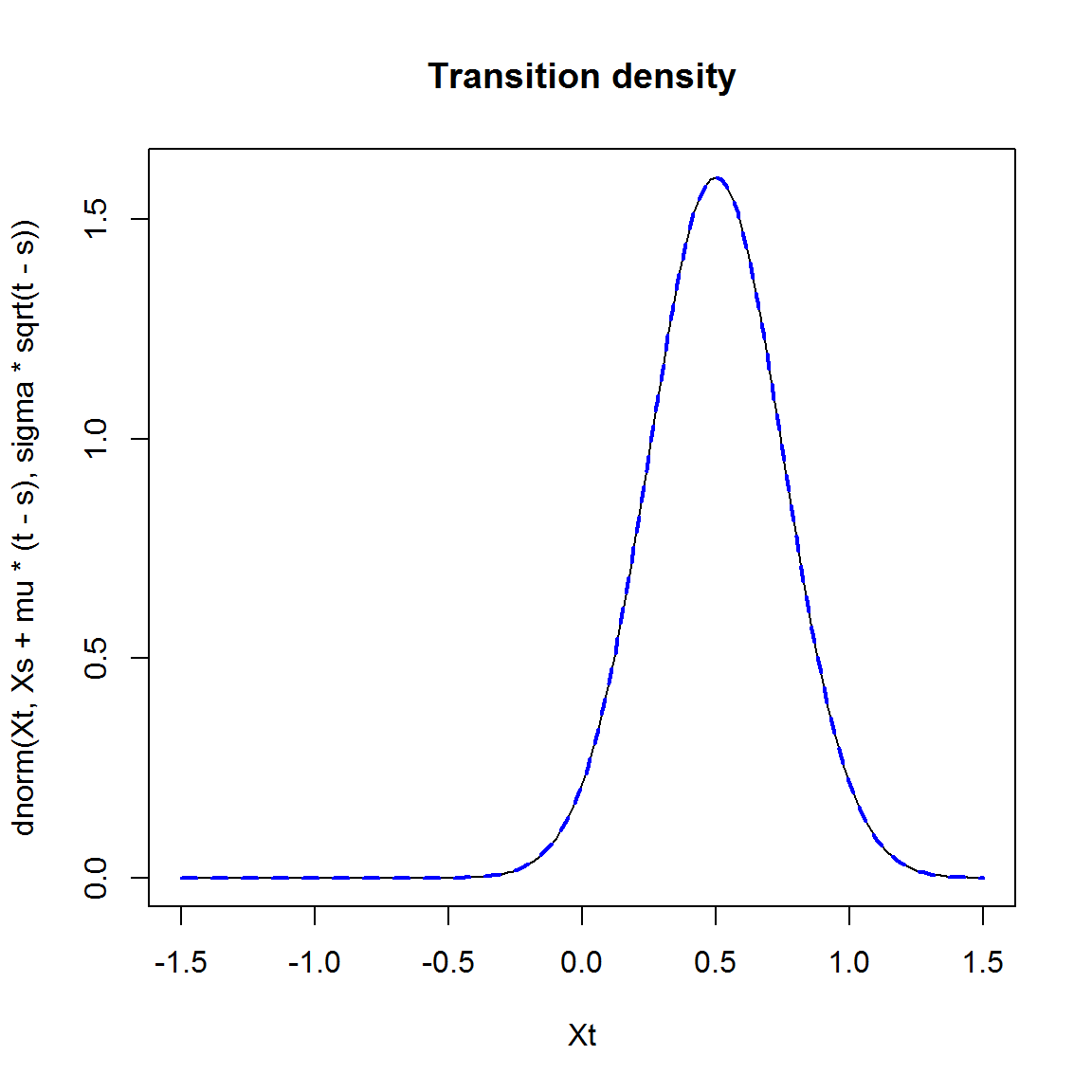Note the call to GQD.remove(). Due to the functional interface, it is possible to have conflicting function names in the workspace. As such GQD.remove() acts as an eraser which cleans the workspace of any existing models or function names that might clash with the pre-defined names listed for DiffusionRgqd. Note also the index [,100]: GQD.density() evaluates the transition density from the initial time up to and including the final transition horizon using a specified step-size. In this case the default increment of 1/100 is used.

# CIR process

Using DiffusionRgqd it is easy to generate the transitional densities of well known diffusion models nested within the GQD framework. Consider a CIR process with SDE:

$dX_t = a(b-X_t)dt+\sigma\sqrt{X_t}dB_t.$

# Remove any existing coefficients
GQD.remove()         
##  "Removed :  G0 Q0"
a = 0.5; b = 5; sigma = 0.35; # Some parameter values.

# Define drift Coefficients.
G0 <- function(t){a*b}
G1 <- function(t){-a}
# Define sinusoidal diffusion coefficient.
Q1 <- function(t){sigma^2}

states     <-  seq(1, 9, 1/10)# State values
initial    <-  6              # Starting value of the process
Tmax       <-  5              # Time horizon
Tstart     <-  1              # Time starts at 1
increment  <-  1/100          # Incremental time steps

# Generate the transitional density
M <- GQD.density(Xs = initial, Xt = states, s = Tstart, t = Tmax, delt = increment)
##
##  ================================================================
##  ================================================================
##  _____________________ Drift Coefficients _______________________
##  G0 : a*b
##  G1 : -a
##  G2
##  ___________________ Diffusion Coefficients _____________________
##  Q0
##  Q1 : sigma^2
##  Q2
##  __________________ Distribution Approximant ____________________
##  P               :
##  alpha           :
##  Trunc. Order    : 4
##  Dens.  Order    : 4
## =================================================================

In order to visualize the density approximation, we can use standard R perspective plots:

persp(x = M$Xt, y = M$time, z = M$density, col = 'white', xlab = 'State (X_t)',ylab = 'Time (t)', zlab = 'Density f(X_t|X_s)', border = NA, shade = 0.5, theta = 145)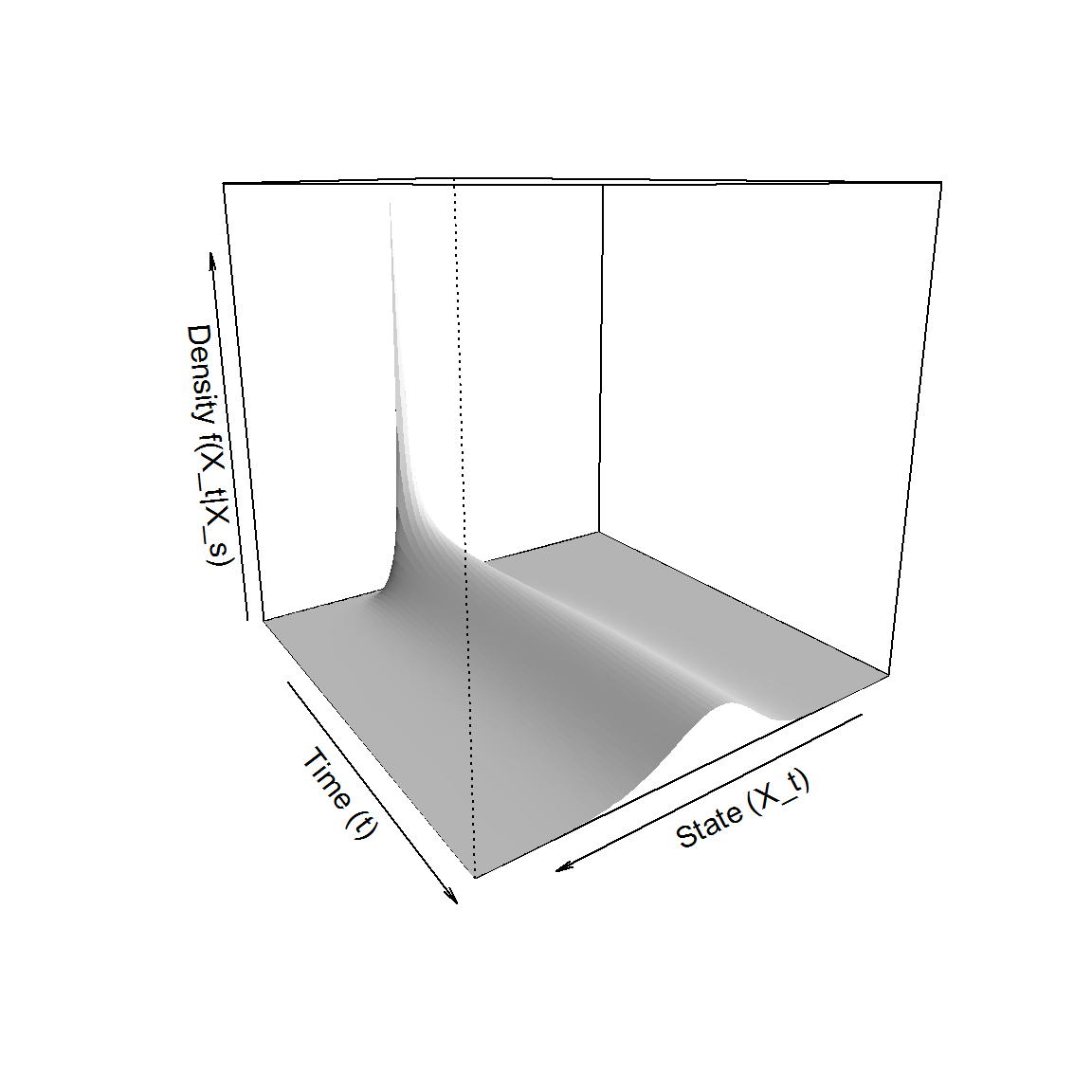Let’s see how the mean of the process evolves over time. In addition to the transitional density GQD.density() returns the cumulant equations used in the calculation of the transitional density. # What is returned: names(M) ##  "density" "Xt" "time" "cumulants" "moments" "mesh" # Plot the mean trajectory: plot(M$cumulants[1,]~M$time, col = '#222299', main = 'Mean trajectory', type = 'l')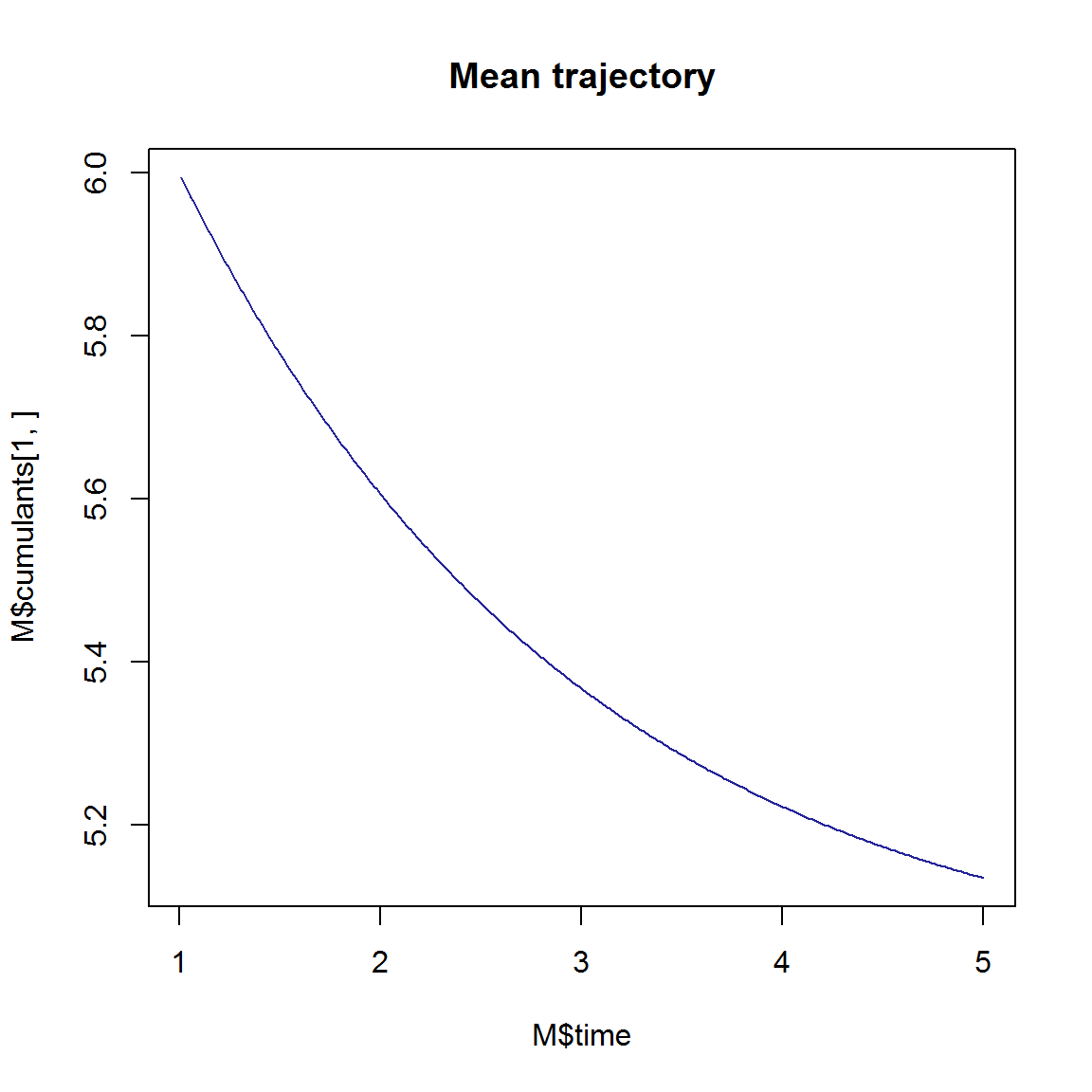And how about the variance of the process? # Plot the variance trajectory: plot(M$cumulants[2,]~M\$time, col = '#222299', main = 'Variance trajectory', type  = 'l')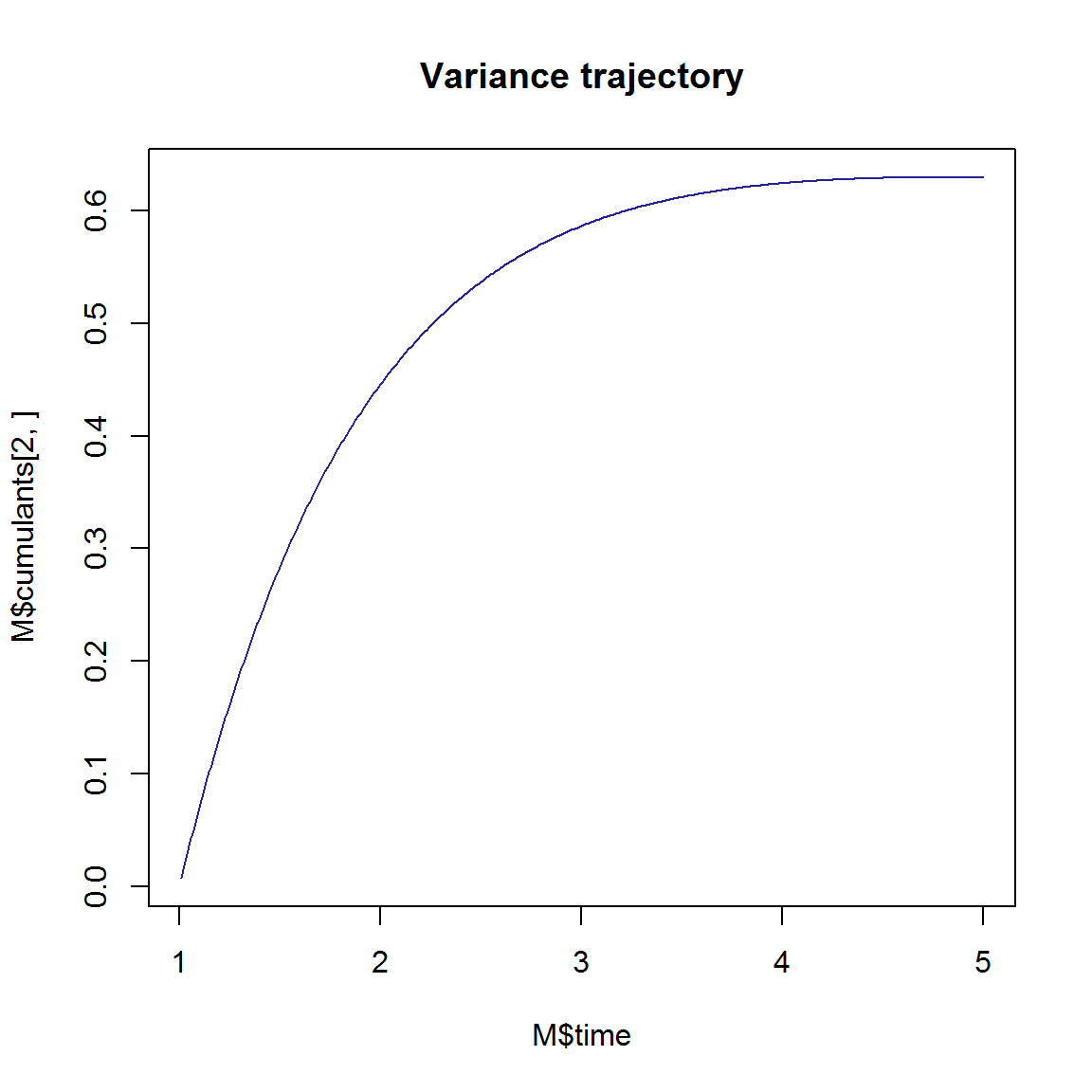browseVignettes('DiffusionRgqd')